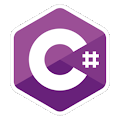Programming Course in C# ¡Free!

# Function returning a value

Proposed exercise

Create a program whose Main must be like this:

public static void Main()
{
int x= 3;
int y = 5;
Console.WriteLine( Sum(x,y) );
}

"Sum" is a function that you must define and that will be called from inside Main. As you can see in the example, it must accept two integers as parameters, and it must return an integer number (the sum of those two numbers).

Output

Solution

```using System;
public class FunctionSum
{
public static int Sum(int a, int b)
{
int result;
result = a + b;
return result;
}

public static void Main()
{
int x= 3;
int y = 5;

Console.WriteLine( Sum(x,y) );
}
}

```